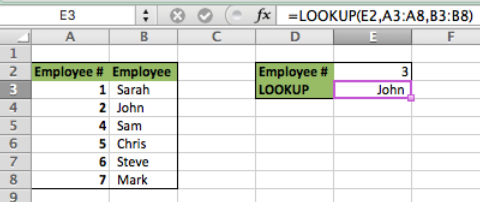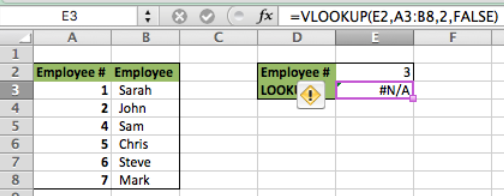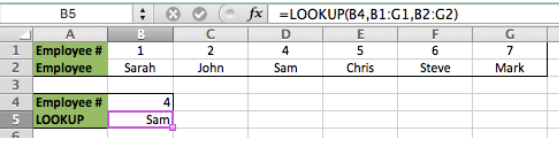Get instant live expert help with Excel or Google Sheets“My Excelchat expert helped me in less than 20 minutes, saving me what would have been 5 hours of work!”

#### Post your problem and you’ll get Expert help in seconds.

Your message must be at least 40 characters
Our professional Expert are available now. Your privacy is guaranteed.

# VLOOKUP vs. LOOKUP: How Are They Different?

The main difference between VLOOKUP and LOOKUP functions is the VLOOKUP is limited to vertical lookups only and the LOOKUP function has cross functionality which means that it can perform both vertical lookups and horizontal lookups. However, there are some other differences as well. We will step through some Examples below to express the differences.

## LOOKUP is limited to Approximate match

• VLOOKUP syntax: `VLOOKUP(Lookup Value, Table Array, Column to Return, Approximate Match [True/False])`
• LOOKUP syntax: `LOOKUP(Lookup Value, Lookup Array, Value to Return Array)`
• We can see that the LOOKUP function doesn’t have the True/False Option
• For this Example, setup your data so that Column A is the lookup array and Column B is the Returned Value array
• In cell E2 enter your lookup value
• In cell E3 type this formula `=LOOKUP(E2,A3:A8,B3:B8)`Figure 1. VLOOKUP vs. LOOKUP

We can see in this Example that the formula incorrectly selected “John” because of the default approximate match. Now lets create the same calculation using VLOOKUP

• Click in cell E3 and type this formula `=VLOOKUP(E2,A3:B8,2,FALSE)`Figure 2. VLOOKUP vs. LOOKUP

We can see from the VLOOKUP example that the formula returned an error because there was no exact match for  “3” in the lookup array

## LOOKUP is Cross-Functional

• In the previous LOOKUP Example, we identified the vertical lookup capabilities of the LOOKUP function. It can also perform a lookup horizontally
• For this example, we will take the same data and assume it is organized horizontally
• In ROW 1 enter the lookup array, enter the return array in ROW 2
• Type “4” in cell B4
• In cell B5 type this formula `=LOOKUP(B4,B1:G1,B2:G2)`Figure 3. VLOOKUP vs. LOOKUP

We can see in this example that the LOOKUP function returns the accurate information from the table even though it is organized horizontally

Solution examplesWhen i do a VLOOKUP, I cannot autofill the formula for the rest of the table. It copies the initial cell that the VLOOKUP was done.
Solved by G. A. in 20 minsI need a formula (I think VLOOKUP) that will enable me to: Use data from one column, compare with another and tell me the difference. Im a teacher and want to work out the amount of marks needed to the next grade boundary. I've created the rest, but this has me stumped!
Solved by F. A. in 28 minshow do I do the same formula for different cells, but i want a different cells value in each of the formulas. For example, if i was trying to find the percentage grade of each student in a class of 300.
Solved by I. W. in 14 minsI need help with a VLOOKUP formula. How do create a formula.
Solved by K. A. in 20 minsI need to copy a VLOOKUP formula to a cell underneath but instead of moving the third value (B55) to (B56) which happens automatically, I want the formula to move from (B55) to (C55). Basically moving to the next column, not the next row. How do I make this happen?
Solved by O. U. in 33 mins